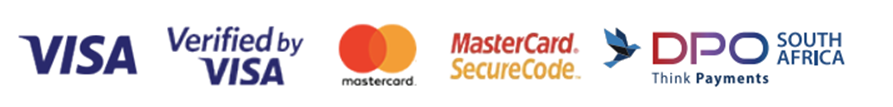﻿ Singapore Maths Preschool - Singapore Maths
Singapore Maths Preschool

Earlybird Kindergarten Mathematics Books 1A, 1B, 2A & 2B are specifically designed in preparation for Grade 1.

Showing 1 - 4 of 4 items
• ##### New Earlybird Kindergarten Mathematics K1A
R140.00

The New Earlybird Kindergarten Mathematics provides a systematic and focused introduction to essential mathematical concepts and basic reasoning skills. There are 4 books in this series K1A; K1B; K2A; K2B.

• ##### New Earlybird Kindergarten Mathematics K1B
R140.00

The New Earlybird Kindergarten Mathematics provides a systematic and focused introduction to essential mathematical concepts and basic reasoning skills. There are 4 books in this series K1A; K1B; K2A; K2B.

• ##### New Earlybird Kindergarten Mathematics K2A
R140.00

The New Earlybird Kindergarten Mathematics provides a systematic and focused introduction to essential mathematical concepts and basic reasoning skills. There are 4 books in this series K1A; K1B; K2A; K2B.

• ##### New Earlybird Kindergarten Mathematics K2B
R140.00

The New Earlybird Kindergarten Mathematics provides a systematic and focused introduction to essential mathematical concepts and basic reasoning skills. There are 4 books in this series K1A; K1B; K2A; K2B.

Showing 1 - 4 of 4 items

#### Categories